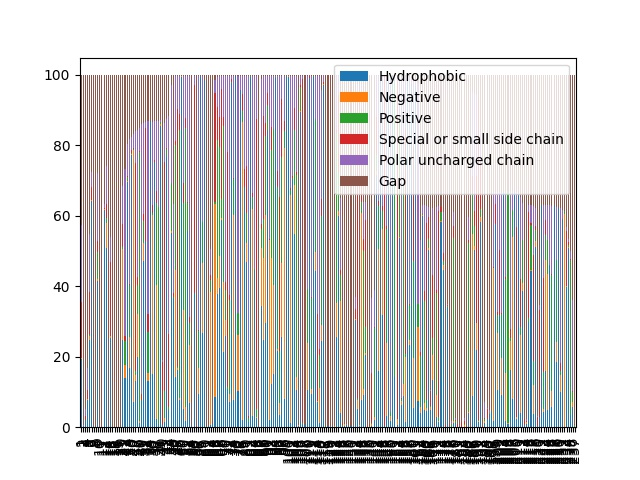# Overlap of bar charts

Hello everyone,

I have a question regarding the visualization of a bar plot that I did with matplotlib.The code that I used to do this figure was:

`transpose_df.plot.bar(stacked=True)`

The` transpose_df` is a dataframe that I created using an empty numpy matrix and then iterate in a database to fill it.

How can I do to fit the figure so there is no overlaping of the values of the x-axis and also the bar are clear.

Thank you.

What’s the shape of your data and how many bars do you intend to have? Increase the figsize might help, something like `df.plot.bar(figsize=(20,5))`